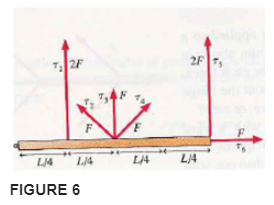# Problem: Six forces, each of magnitude either F or 2F, are applied to a door as seen from above in Figure 6. Rank in order, from smallest to largest, the six torques τ1 to τ6 about the hinge.

###### FREE Expert Solution

Torque:

$\overline{){\mathbf{\tau }}{\mathbf{=}}{\mathbf{r}}{\mathbf{·}}{\mathbf{F}}{\mathbf{s}}{\mathbf{i}}{\mathbf{n}}{\mathbf{\theta }}}$

When r and F are perpendicular, θ = 90°. Whe r and F are parallel, θ = 0°.

τ1 = r•Fsinθ = (L/4)(2F)(sin 90) = (LF)/2

τ2 = r•Fsinθ = (L/2)(F)(sinθ2) = (LFsinθ2)/2 or (LF/2)sinθ2

89% (398 ratings)###### Problem Details

Six forces, each of magnitude either or 2F, are applied to a door as seen from above in Figure 6. Rank in order, from smallest to largest, the six torques τ1 to τ6 about the hinge.